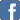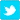Astronomical Unit

Posted on the 25 March 2011 by Gabe12logan
Astronomical unit (AU) is a unit of length. It is used in astronomy, and is approximately equal to the distance of Earth from the Sun. Currently accepted value is equal to 149 597 870 691 (about 150 million kilometers).Astronomical unit is the radius of the circular path along which the body of negligible mass and that is not subject to perturbations would orbited the Sun with a period of 2p / k days, where k is the Gaussian gravitational constant. Astronomical unit is slightly smaller amount of large axis of the Earth's path around the Sun, so we can say that this is the mean distance of Earth from the Sun.This unit is very convenient for expressing distances in the Solar System. For example the diameter of the orbit of the farthest planet in our solar system is about 80 AU.
It is used often in astronomy among planetary distances, within the Solar System and other planetary systems. For interstellar distances are used larger units, light years and parsec.